# NCERT Solutions class-11 Maths Exercise 12.3## myCBSEguide App

CBSE, NCERT, JEE Main, NEET-UG, NDA, Exam Papers, Question Bank, NCERT Solutions, Exemplars, Revision Notes, Free Videos, MCQ Tests & more.

Exercise 12.3

1. Find the coordinates of the point which divides the line segment joining the points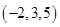and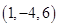in the ratio:

(i) 2: 3 internally,

(ii) 2: 3 externally.

Ans. (i) Let P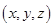be any point which divides the line segment joining points Aand Bin the ratio 2: 3 internally. Then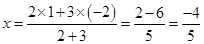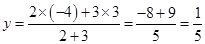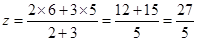Therefore, Coordinates of P are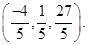(ii) Let Pbe any point which divides the line segment joining points Aand Bin the ratio 2: 3 externally. Then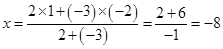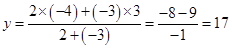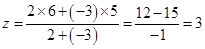Therefore, Coordinates of P are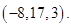2. Given that P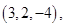Q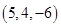and R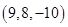are collinear. Find the ratio in which Q divides PR.

Ans. Let Qdivides the line segment joining points P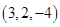and Rin the ratio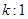internally. Then

Coordinates of Q are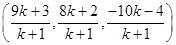According to question,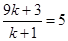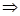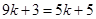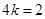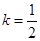Therefore, Q divides the line segment joining the points P and R in the ratio 1: 2.

3. Find the ratio in which the YZ-plane divides the line segment formed by joining the points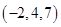and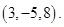Ans. Let the line segment joining points Aand B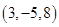be divided by YZ-plane at a point C in the ratiointernally. Then

Coordinates of C are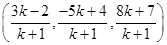According to question, C lies on YZ-plane, i.e.,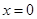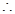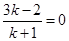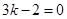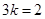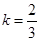Therefore, the required ratio is 2: 3.

4. Using section formula, show that the points A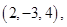B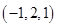and C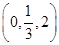are collinear.

Ans. Let the points Bdivides the line segment joining A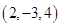and Cin the ratiointernally.

Then coordinates of B =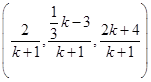Now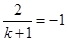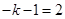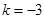5. Find the coordinates of the points which trisect the line segment joining the points P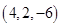and Q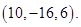Ans. Let R and S be two points which trisect the line segment PQ.

Then PR = RS = SQPoint R divides the lines segment PQ in the ratio 1: 2.

Then Coordinates of R =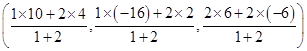=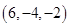Again, Point S divides the line segment PQ in the ratio 2: 1.

Then Coordinates of S =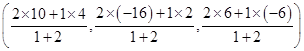=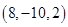### 9 thoughts on “NCERT Solutions class-11 Maths Exercise 12.3”

1. Q4 of ex 12.3 is not solved tatally. It’s solution is half way done.

2. I like it as it is very helpful

3. Nice Work Good Explanation Well Done I Like This Because it’s Given in a very simple concept EXCELLENT????

4. Nice Work Good Explanation Well Done I Like This Because it’s Given in a very simple concept EXCELLENT????

5. It’s Very useful for me really

6. This site is very helpful for students

7. Thank you very much

8. Nice

9. Solutions are very handy IN school exam